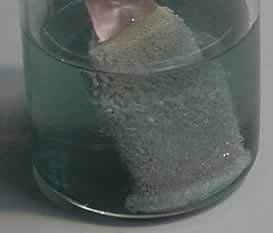Preparatory

chemistryLearning Outcomes

Students should be able to:

 Chemical formulae -name the precipitate formed when two known solutions are mixed. Link -write the correct formula of an ionic compound given its name and valency table. Link -name an ionic compound given its formula. Link -interpret a formula , identify the elements present and the number of atoms of each element. Link -calculate the formula mass of a substance given its formula. Link -use the covalency of atoms and the Octet rule to predict molecular formula and even molecular shapes using this applet Link -draw Lewis dot structures of molecules Link Chemical equations -write an overall word equation for a precipitation reaction given the two reacting solutions. Link -explain the "Law of Conservation of Mass" as it applies to chemical reactions. Link -write balanced chemical equations for simple reactions. Link -construct balanced chemical equations for precipitate reactions Link -write the chemical equations for reactions between acids and: * carbonates * metal oxides * metals Link Atomic structure and bonding -describe the structure of the atom using the terms electrons, protons, neutrons, nucleus and energy levels. Link -describe the arrangement of electrons around the nucleus of a given element. Link -identify a stable electronic arrangement Link -predict the charge of ions formed by electron transfer. Link -outline the two ways in which electrons are transferred between atoms. Link -predict if a covalent or ionic bond will form between two atoms and give the formula of the product formed. Link Chemical reactions around us: -describe several chemical reactions that occur around the home, by identifying the reactants, the products and any special conditions required to make them proceed. Link -explain,using the collision theory, why activation energy is needed to start some reactions. Link Rates of reaction -explain how reactions take place according to the "Collision Theory" Link -explain, in terms of the collision theory, how an increase in the surface area of the reactants results in an increase in the rate of a reaction Link -explain, in terms of the collision theory, how an increase in the concentration of the reactants results in an increase in the rate of a reaction Link -.explain, in terms of the collision theory, how an increase in heat results in an increase in the rate of a reaction Link -explain how a catalyst increases the rate of reaction. Link -explain, using the term activation energy, why a flame is needed to light a gas oven. Link -perform experiments, collect, interpret data and write proper scientific reports. Link The Mole -describe the mole as a unit not too dissimilar to the dozen having the number 6 X 1023 rather than 12. Link -perform calculations to obtain the number of mole present in a given mass of pure substance. Link -perform calculations to convert mole of substance to number of particles. Link -perform calculations to convert mole of substance to mass Link -derive the number of mole of a substance given its mass. Link -perform simple experiments to calculate the mole of product and reactant. Link -perform a simple experiment to calculate the mole of water molecules present in a given mass of copper sulfate Link Simple stoichiometry -outline the similarities between a chemical equation and a recipe giving simple examples. Link -explain what a chemical equation tells us Link -given a balanced equation, give the ratio in which atoms of different reactants must be supplied and the ratio in which products are formed. Link -given the amount of one of the products or reactants in a chemical equation, calculate the amount of any reactant needed or product formed. Link Conduct a simple experiment to determine the mass of reactants needed to produce a known mass of product. Link Periodic table -describe how elements are arranged in the periodic table according to their atomic number. Link -recall that the periodic table is set out in columns(groups) and rows(periods) Link -recall that the number of the row indicates the last energy level to be occupied by electrons. Link -recall that the number of the group(column) indicates the number of electrons in the last energy level. Link -given the atomic number of an element place it in its correct position in the periodic table. Link Poster on the uses of chemistry in society using plastics The poster should address - some diverse uses of plastics and the properties of plastics that have allowed them to be used in these ways. - identify one plastic that is widely used and discuss its uses and the properties that allow it to be used in this way. - explain how it is moulded and its ability to be recycled - discuss the chemical bonding, including, branching and crosslinking, that gives your chosen plastic its different properties. - explain why the selection of a monomer is important to the physical properties of a plastic. Give two examples one of a thermosetting plastic and the other of a thermoplastic. Link

Demonstrations
The serpent as a demonstration of use of a catalyst
Making money increase in value.
The bouncing ball
Reactivity of sodium in water
Oxygen gas igniting a candle.
Rusting of iron in oxygen. Demonstration of increasing the rate of the rusting reaction when heat is applied.

Activities
Recovery of copper (conservation of mass)
Recovery of copper metal from copper carbonate
Conservation of mass(recovery of copper)
Making sparklers Reaction rate and surface area
Precipitation reactions
Rates of reaction
Making a home battery.
Mole ratios:
-Copper in copper carbonate
-Water in hydrated copper sulfate
-Magnesium oxide

Revision tests

Bonding
Atomic structure
Isotopes
Periodic table
Chemical equations and ionic formulae
. Note when attempting this test that a precipitate is an insoluble solid and has the subfix (s). Before attempting this test it is best to refresh yourself with precipitates.
Particle theory